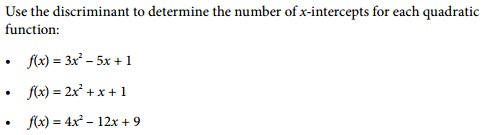# Functions:using discriminant to find number of x-intercepts

Evangeline101

## Homework Statement## Homework Equations

Discriminant: b2 - 4ac

## The Attempt at a Solution

a) [/B]f(x) = 3x2 – 5x + 1

y = 3x2 – 5x +1

Substitute a=3, b= -5, c=1 into the discriminant:

b2 – 4ac = (-5)2 – 4(3)(1)

=25 – 12

= 13 This number is positive.

Since b2 – 4ac > 0, there are two real roots, so the quadrative function has two x-intercepts.

b) f(x) = 2x2 + x +1

y = 2x2 + x +1

Substitute a=2, b=1, c=1 into the discriminant:

b2 – 4ac = (1)2 – 4(2)(1)

= 1 – 8

= -7 This number is negative.

Since b2 – 4ac < 0, there are no real roots, so the quadratic function does not have any x-intercepts.

c) f(x) = 4x2 – 12x + 9

y = 4x2 – 12x + 9

Substitute a=4, b= -12, c=9 into the discriminant:

b2 – 4ac = (-12)2 – 4(4)(9)

=144 – 144

= 0

Since b2 – 4ac = 0, there is one (double) real root, so the quadratic function has one x-intercept.

Is this correct?

Mastermind01
This is absolutely correct. You can additionally verify by drawing the graphs for the equations.

Evangeline101
Okay. Thanks for verifying my answerMastermind01
Okay. Thanks for verifying my answerYou are welcome. However you could have done it yourself by hand-drawing the graph or using a graphing calculator.

Evangeline101
However you could have done it yourself by hand-drawing the graph or using a graphing calculator.

Oh okay. But this is the way they taught me in the lesson, they did not ask me to graph anything.

Mastermind01
Oh okay. But this is the way they taught me in the lesson, they did not ask me to graph anything.